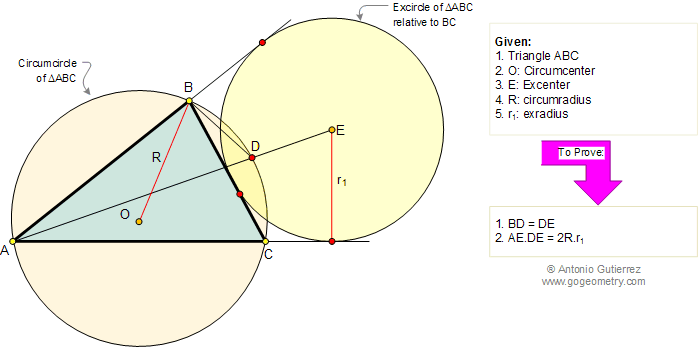Problem 156. Triangle, Circumradius, Exradius, Chord, Secant line
 In the figure below, ABC is a triangle inscribed in a circle of center O (circumcenter) and E is the center (excenter) of the excircle relative to side BC. Line AE and the circumcircle meet at D. If R is the circumradius and r1 is the exradius (radius of circle E), (1) prove that BD = DE, and (2) prove that AE.DE = 2R.r1. View or post a solution.Home | Geometry | Search | Wolfram Demonstrations Project | Problems | 151-160 | Email | By Antonio Gutierrez